In :
from thinkbayes import Pmf

In :
pmf = Pmf()
for x in range(1, 7):
pmf.Set(x, 1)

In :
pmf.Print()

1 1
2 1
3 1
4 1
5 1
6 1

In :
pmf.Normalize()

Out:
6
In :
pmf.Print()

1 0.166666666667
2 0.166666666667
3 0.166666666667
4 0.166666666667
5 0.166666666667
6 0.166666666667


## Prior¶

In :
pmf = Pmf()
pmf.Set('Bowl 1', 0.5)
pmf.Set('Bowl 2', 0.5)


## Update¶

P(Vanilla | Bowl 1) = 3/4

P(Vanilla | Bowl 1) = 2/4

In :
pmf.Mult('Bowl 1', 0.75)
pmf.Mult('Bowl 2', 0.5)


## Normalize¶

In :
pmf.Normalize()
print pmf.Prob('Bowl 1')

0.6


## The dice problem¶

We representa box with dice of [4, 6, 8, 12, 20] sides.

In :
from thinkbayes import Suite

suite = Suite([4, 6, 8, 12, 20])
suite.Print()

4 0.2
6 0.2
8 0.2
12 0.2
20 0.2


We're going to add a Likelihood method

In :
from thinkbayes import Suite

class Dice(Suite):
def Likelihood(self, hypo, data):
"""Computes the likelihood of the data under the hypothesis.

hypo: integer number of sides on the die
data: integer die roll
"""
num_sides = hypo
outcome = data

if outcome > num_sides:
return 0
else:
return 1.0/num_sides


Let's build a suite that represents our dice:

In :
suite = Dice([4, 6, 8, 12, 20])


And now let's say that we roll once and get a 6. What does this tells us about the probabilities for the various dice?

In :
suite.Update(6)
print 'After one 6'
suite.Print()

After one 6
4 0.0
6 0.392156862745
8 0.294117647059
12 0.196078431373
20 0.117647058824


And now let's roll a few more dice and figure out how that informs us about the probability of the die being of a specific size:

In :
for roll in [6, 4, 8, 7, 7, 2]:
suite.Update(roll)

suite.Print()

4 0.0
6 0.0
8 0.943248453672
12 0.0552061280613
20 0.0015454182665


## The German Tank problem¶

In :
%pylab inline

Welcome to pylab, a matplotlib-based Python environment [backend: module://IPython.kernel.zmq.pylab.backend_inline].

In :
import thinkbayes
import myplot

class Train(thinkbayes.Suite):
"""Suite of hypotheses about the number of trains."""

def Likelihood(self, hypo, data):
"""Computes the likelihood of the data under the hypothesis.

hypo: integer hypothesis about the number of trains
data: integer serial number of the observed train
"""
num_trains = hypo
train_seen = data
if train_seen > num_trains:
return 0
else:
return 1.0/num_trains

Given the data, our mean estimate is: 597.344008084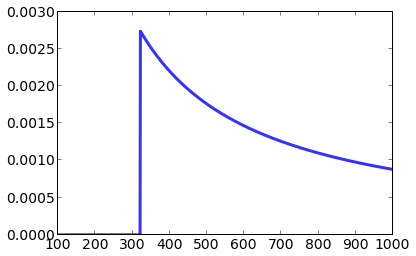In :
hypos = xrange(100, 1001)
suite = Train(hypos)

suite.Update(321)
print 'Given the data, our mean estimate is:',
print suite.Mean()

myplot.Pmf(suite)

Given the data, our mean estimate is: 597.344008084Let's now assume we're spotting a few more trains, and let's see how our posterior updates as we measure this data:

In :
for train in [782, 423]:
suite.Update(train)

print 'Given the data, our mean estimate is:',
print suite.Mean()
myplot.Pmf(suite)

Given the data, our mean estimate is: 877.54322135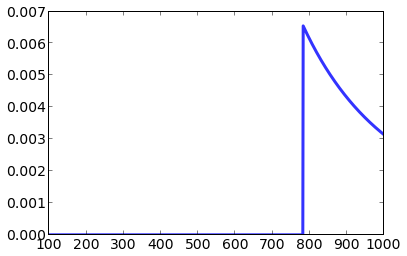## Euro problem¶

Estimation: based on the data (140h, 110t), what is the probability that the coin lands heads?

Hypothesis testing: what is the probability that the coin is fair?

In :
class Euro(thinkbayes.Suite):

def Likelihood(self, hypo, data):
"""Computes the likelihood of the data under the hypothesis.

hypo: integer value of x, the probability of heads (0-100)
data: string 'H' or 'T'
"""
# Note: while we're writing the likelihood function, we
# *assume* the hypothesis is true.  Don't forget this!

# We convert the hypothesis to a probability in the 0-1 range:
x = hypo/100.0

# Now, since the hypothesis is about Heads, if the data is H
# it means the hypothesis is True, so we can just return the hypothesis
if data == 'H':
return x
else:
return 1-x

suite = Euro(range(0, 101))
myplot.Pmf(suite)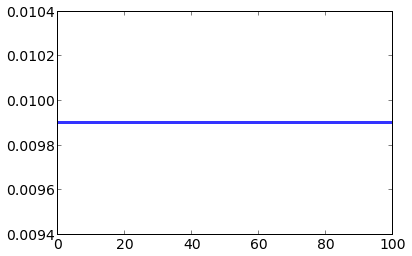In :
suite.Update('H')
myplot.Pmf(suite)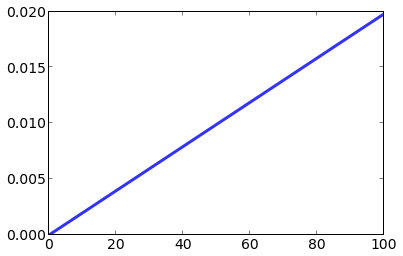In :
suite.Update('H')
myplot.Pmf(suite)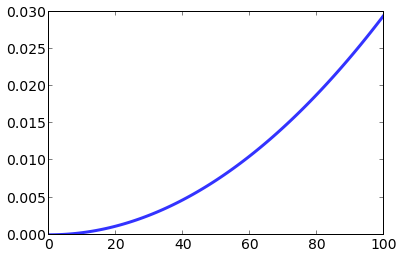In :
suite.Update('T')
myplot.Pmf(suite)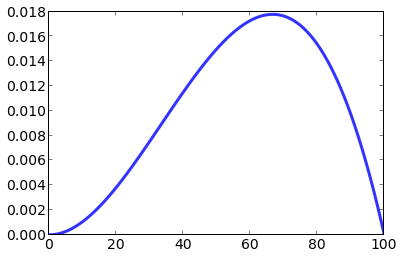In :
for outocme in 'HHHHHHHHTTT':
suite.Update(outocme)
myplot.Pmf(suite)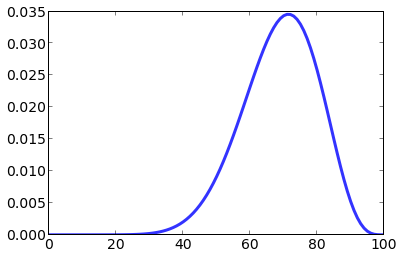In :
suite = Euro(range(0, 101))
for outocme in 'H'*140 + 'T'*110:
suite.Update(outocme)
myplot.Pmf(suite)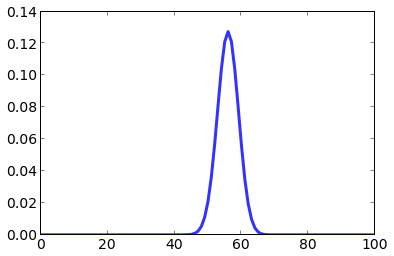What is the probability that the coin is fair? I.e., what is the prob. that x is 50%?

In :
suite.Prob(50)

Out:
0.02097652612954468

## Hypothesis testing¶

In :
%load euro2.py

In :
class Euro(thinkbayes.Suite):

def Likelihood(self, hypo, data):
"""Computes the likelihood of the data under the hypothesis.

hypo: integer value of x, the probability of heads (0-100)
data: string 'H' or 'T'
"""
x = hypo / 100.0
return like

def AverageLikelihood(suite, data):
"""Computes the average likelihood over all hypothesis in suite.

Args:
suite: Suite of hypotheses
data: some representation of the observed data

Returns:
float
"""
total = 0

for hypo, prob in suite.Items():
like = suite.Likelihood(hypo, data)
total += prob * like


In :
fair = Euro()
fair.Set(50, 1)

bias = Euro()
for x in range(0, 101):
if x != 50:
bias.Set(x, 1)
bias.Normalize()

data = 140, 110

like_bias = AverageLikelihood(bias, data)
print 'like_bias', like_bias

like_fair = AverageLikelihood(fair, data)
print 'like_fair', like_fair

ratio = like_bias / like_fair
print 'Bayes factor', ratio

like_bias 2.57964902292e-76
like_fair 5.52714787526e-76
Bayes factor 0.466723359161

In :
bias = Euro()

for x in range(40, 70):
if x != 50:
bias.Set(x, 1)
bias.Normalize()

like_bias = AverageLikelihood(bias, data)
print 'like_bias', like_bias

ratio = like_bias / like_fair
print 'Bayes factor', ratio

like_bias 8.89531486664e-76
Bayes factor 1.60938608255


## SAT scores¶

In :
import csv
import math
import numpy
import sys

import matplotlib

import myplot

"""Reads a CSV file of SAT scales (maps from raw score to standard score).

Args:
filename: string filename

Returns: thinkbayes.Interpolator object
"""
def ParseRange(s):
t = [int(x) for x in s.split('-')]
return 1.0 * sum(t) / len(t)

fp = open(filename)
raws = []
scores = []

try:
raw = int(t[col])
raws.append(raw)
score = ParseRange(t[col+1])
scores.append(score)
except:
pass

raws.sort()
scores.sort()
return thinkbayes.Interpolator(raws, scores)

"""Reads a CSV file of SAT scores.

Args:
filename: string filename

Returns:
list of (score, freq) pairs
"""
fp = open(filename)
res = []

try:
score = int(t)
freq = int(t)
res.append((score, freq))
except ValueError:
pass

return res

def DivideValues(pmf, denom):
"""Divides the values in a Pmf by denom.

Returns a new Pmf.
"""
new = thinkbayes.Pmf()
denom = float(denom)
for val, prob in pmf.Items():
x = val / denom
new.Set(x, prob)
return new

class Exam(object):

Contains the distribution of scaled scores and an
Interpolator that maps between scaled and raw scores.
"""
def __init__(self):

score_pmf = thinkbayes.MakePmfFromDict(dict(scores))

self.raw = self.ReverseScale(score_pmf)
self.max_score = max(self.raw.Values())
self.prior = DivideValues(self.raw, denom=self.max_score)

def Lookup(self, raw):
"""Looks up a raw score and returns a scaled score."""
return self.scale.Lookup(raw)

def Reverse(self, score):
"""Looks up a scaled score and returns a raw score.

Since we ignore the penalty, negative scores round up to zero.
"""
raw = self.scale.Reverse(score)
return raw if raw > 0 else 0

def ReverseScale(self, pmf):
"""Applies the reverse scale to the values of a PMF.

Args:
pmf: Pmf object
scale: Interpolator object

Returns:
new Pmf
"""
new = thinkbayes.Pmf()
for val, prob in pmf.Items():
raw = self.Reverse(val)
new.Incr(raw, prob)
return new

class Sat(thinkbayes.Suite):
"""Represents the distribution of efficacy for a test-taker."""

def __init__(self, exam):
thinkbayes.Suite.__init__(self)

self.exam = exam

for p, prob in exam.prior.Items():
self.Set(p, prob)

def Likelihood(self, hypo, data):
"""Computes the likelihood of a test score, given efficacy."""
p = hypo
score = data
raw = self.exam.Reverse(score)

yes, no = raw, self.exam.max_score - raw
like = p**yes * (1-p)**no
return like

def MakeFigures(exam, alice, bob):
formats=['png']

myplot.Pmf(exam.prior, label='prior')
myplot.Save(root='sat_prior',
formats=formats,
xlabel='p',
ylabel='PMF')

myplot.Clf()
myplot.Pmfs([alice, bob])
myplot.Save(root='sat_posterior',
formats=formats,
xlabel='p',
ylabel='PMF')

In :
def PmfProbGreater(pmf1, pmf2):
"""Probability that a value from pmf1 is more than a value from pmf2.

Args:
pmf1: Pmf object
pmf2: Pmf object

Returns:
float probability
"""
total = 0.0
for v1, p1 in pmf1.Items():
for v2, p2 in pmf2.Items():
if p1 > p2:
total += p1*p2

exam = Exam()

alice = Sat(exam)
alice.name = 'alice'
alice.Update(780)

bob = Sat(exam)
bob.name = 'bob'
bob.Update(760)

print 'Prob Alice is "smarter":', PmfProbGreater(alice, bob)

Prob Alice is "smarter": 0.572030956616

In [ ]: